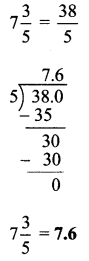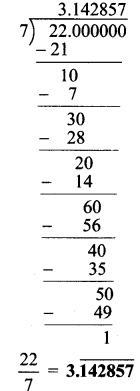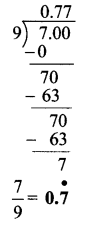# Maharashtra Board Practice Set 24 Class 7 Maths Solutions Chapter 5 Operations on Rational Numbers

Balbharti Maharashtra State Board Class 7 Maths Solutions covers the 7th Std Maths Practice Set 24 Answers Solutions Chapter 5 Operations on Rational Numbers.

## Operations on Rational Numbers Class 7 Practice Set 24 Answers Solutions Chapter 5

Question 1.
Write the following rational numbers in decimal form.
i. $$\frac { 13 }{ 4 }$$
ii. $$\frac { -7 }{ 8 }$$
iii. $$7\frac { 3 }{ 5 }$$
iv. $$\frac { 5 }{ 12 }$$
v. $$\frac { 22 }{ 7 }$$
vi. $$\frac { 4 }{ 3 }$$
vii. $$\frac { 7 }{ 9 }$$
Solution:
i. $$\frac { 13 }{ 4 }$$ii. $$\frac { -7 }{ 8 }$$iii. $$7\frac { 3 }{ 5 }$$iv. $$\frac { 5 }{ 12 }$$v. $$\frac { 22 }{ 7 }$$vi. $$\frac { 4 }{ 3 }$$vii. $$\frac { 7 }{ 9 }$$Maharashtra Board Class 7 Maths Chapter 5 Operations on Rational Numbers Practice Set 24 Intext Questions and Activities

Question 1.
Without using division, can we tell from the denominator of a fraction, whether the decimal form of the fraction will be a terminating decimal? Find out. (Textbook pg. no. 40)
Solution:
If the prime factorization of the denominator of a fraction has only factors as 2 or 5 or a combination of 2 and 5 then the decimal form of that fractional will be a terminating decimal form.
Consider the fractions $$\frac { 17 }{ 20 }$$ and $$\frac { 19 }{ 6 }$$
Now, 20 = 2 x 2 x 5, and 6 = 2 x 3
∴ $$\frac { 17 }{ 20 }$$ is terminating decimal form while $$\frac { 19 }{ 6 }$$ is recurring decimal form.